Question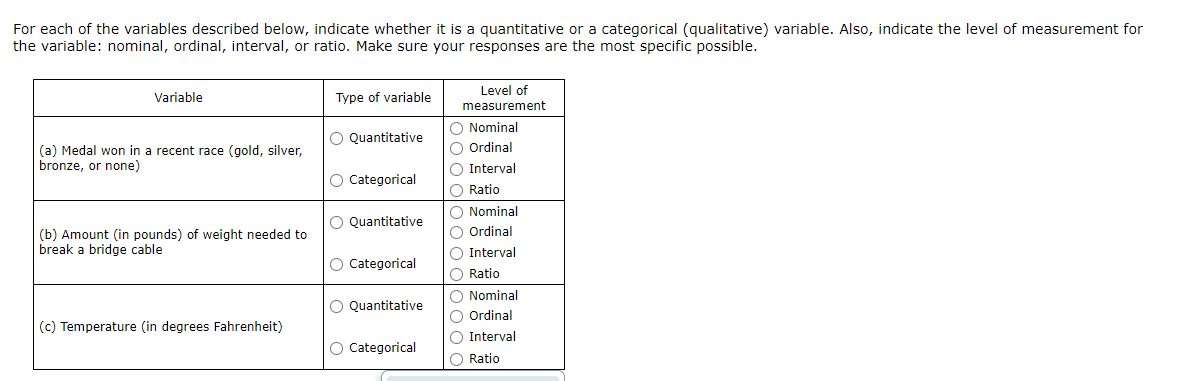• Medal won in a recent race:
Here, the Medal won refers to the type of Medal won - Gold, Silver, Bronze etc. It describes the quality or characteristics of the Medal. Hence the medals are categorical and not Quantitative, as the data does not include numbers.
Also, the medals can be ordered. That is, Gold is best, followed by Silver and then Bronze. Hence, the variable is Ordinal. Hence, the correct option is:
Categorical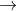Ordinal .
• Amount (in pound) of weight needed to break a bridge cable:
Here, the data is numbered. That is, the variable is the quantity of weight required. Hence, this is Quantitative data.
Also, a weight of 0 indicates the absence of any weight and is not just "any other" value. This is a characteristics of ratio scale. Hence, the correct option is:
QuantitativeRatio .
• Temperature (in degree Farenheit)
Here, the data is numbered. That is, the variable is the temperature, indicating the degree of hotness or coldness. Also, this type of data cannot be categorised. Hence, the data is quatitative in nature.
Also, a temperature of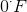does not have any significance. It is just like any other temperature values.does not mean the absence of temperature. This is a characteristic of Interval scale. Hence, the correct option is:
QuantitativeInterval .

I hope this clarifies your doubt. If you're satisfied with the solution, hit the Like button. For further clarification, comment below. Thank You. :)

Close price (in dollars) of a stock

#### Earn Coins

Coins can be redeemed for fabulous gifts.

Similar Homework Help Questions
• ### For each of the variables described below, indicate whether it is a quantitative or a categorical...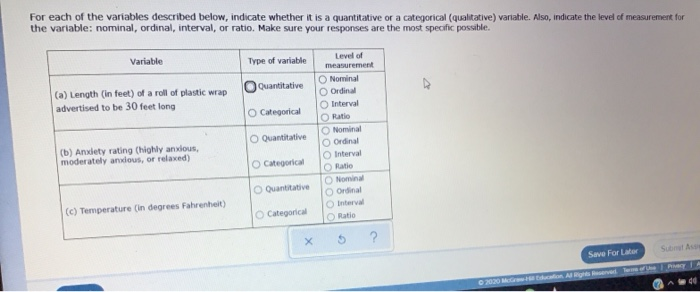For each of the variables described below, indicate whether it is a quantitative or a categorical (qualitative) variable. Also, indicate the level of measurement for the variable: nominal, ordinal, interval, or ratio. Make sure your responses are the most specific possible. Variable Type of variable Quantitative (a) Length (in feet) of a roll of plastic wrap advertised to be 30 feet long O Categorical Quantitative Level of measurement Nominal Ordinal Interval O Ratio Nominal Ordinal Interval Ratio Nominal Ordinal Inter...

• ### For each of the variables described below, indicate whether it is a quantitative or a categorical...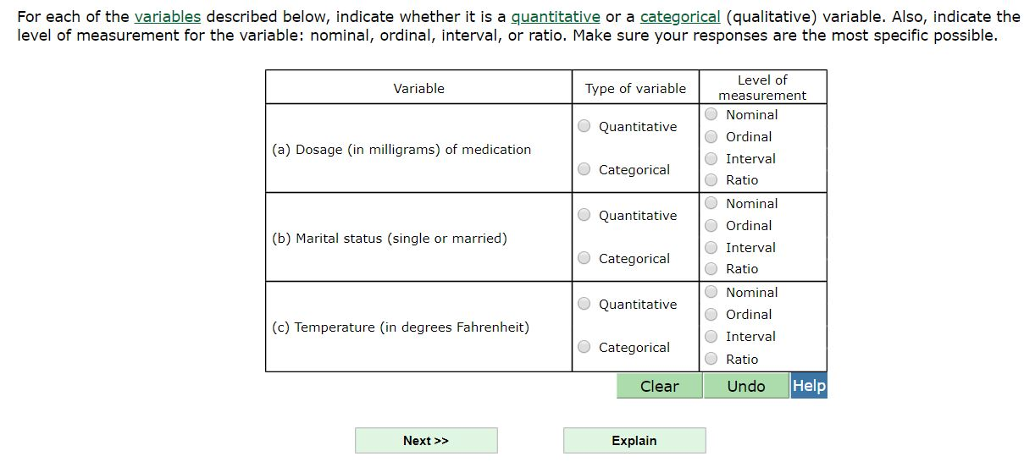For each of the variables described below, indicate whether it is a quantitative or a categorical (qualitative) variable. Also, indicate the level of measurement for the variable: nominal, ordinal, interval, or ratio. Make sure your responses are the most specific possible Level of measurement Nominal Ordinal Interval Ratio Variable Type of variable Quantitative Categorical O Quantitative Categorical O Quantitative (a) Dosage (in milligrams) of medication O Nominal O Ordinal O Interval O Ratio (b) Marital status (single or married) Nominal...

• ### For each of the variables described below, indicate whether it is a quantitative or a categorical...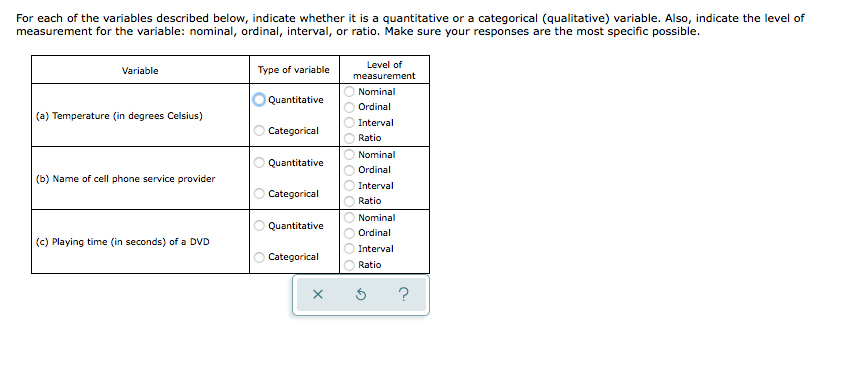For each of the variables described below, indicate whether it is a quantitative or a categorical (qualitative) variable. Also, indicate the level of measurement for the variable: nominal, ordinal, interval, or ratio. Make sure your responses are the most specific possible. Variable Type of variable Quantitative (a) Temperature (in degrees Celsius) Categorical Quantitative Level of measurement Nominal Ordinal Interval Ratio Nominal Ordinal Interval Ratio Nominal Ordinal Interval Ratio (b) Name of cell phone service provider Categorical OOOOOOOOO Quantitative (c) Playing...

• ### For each of the variables described below, indicate whether it is a quantitative or a categorical...

For each of the variables described below, indicate whether it is a quantitative or a categorical (qualitative) variable. Also, indicate the level of measurement for the variable: nominal, ordinal, interval, or ratio. Make sure your responses are the most specific possible. Variable Type of variable Level of measurement (a) Dosage (in milligrams) of medication Quantitative Nominal Ordinal Categorical Interval Ratio (b) Customer satisfaction rating (very satisfied, somewhat satisfied, somewhat dissatisfied, or very dissatisfied) Quantitative Nominal Ordinal Categorical Interval Ratio (c)...

• ### For each of the variables described below, indicate whether it is a quantitative or a categorical...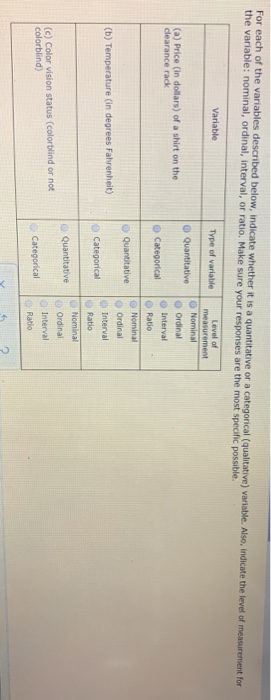For each of the variables described below, indicate whether it is a quantitative or a categorical (qualitative) variable. Also, indicate the level of measurement for the variable: nominal, ordinal, interval, or ratio. Make sure your responses are the most specific possible Variable Type of variable Level of measurement Nominal O Quantitative Ordinal (a) Price (in dollars) of a shirt on the clearance rack Interval Categorical Ratio Quantitative (b) Temperature (in degrees Fahrenheit) Categorical Nominal Ordinal Interval Ratio Nominal Ordinal Interval...

• ### 10 11 For each of the variables described below, indicate whether it is a quantitative or...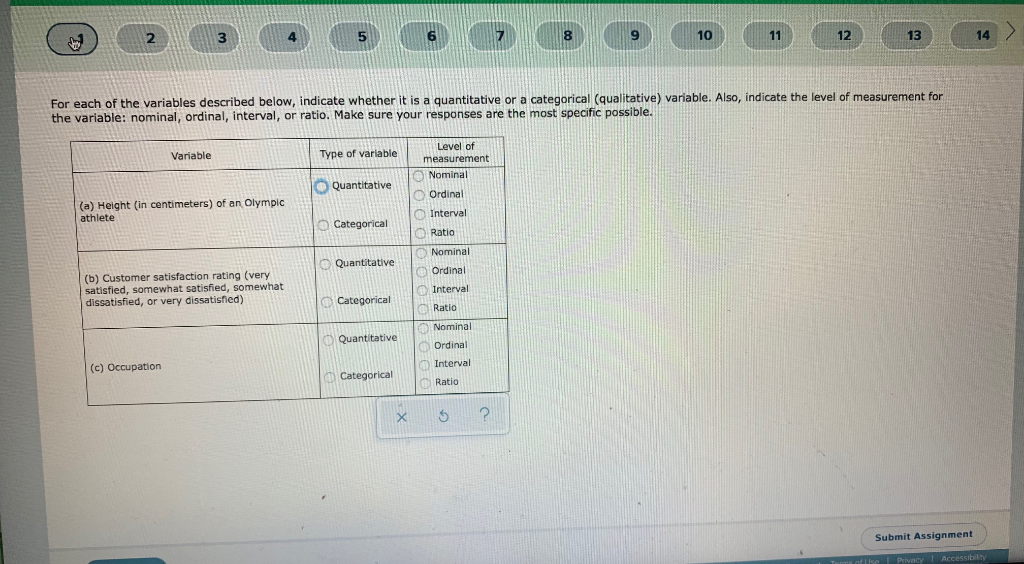10 11 For each of the variables described below, indicate whether it is a quantitative or a categorical (qualitative) variable. Also, indicate the level of measurement for the variable: nominal, ordinal, interval, or ratio. Make sure your responses are the most specific possible. Variable Type of variable Level of measurement Nominal Ordinal Quantitative (a) Height (in centimeters) of an Olympic athlete Categorical Interval Ratio Quantitative Nominal Ordinal (b) Customer satisfaction rating (very satisfied, somewhat satisfied, somewhat dissatisfied, or very dissatisfied)...

• ### Classify the following data. Indicate whether the data is qualitative or quantitative, indicate whether the data...

Classify the following data. Indicate whether the data is qualitative or quantitative, indicate whether the data is discrete, continuous, or neither, and indicate the level of measurement for the data. A survey response to "Do you favor a flat tax?" 1) Yes 2) No 1. Are these data qualitative or quantitative? 2. Are these data discrete, continuous or neither? 3. What is the highest level of measurement the data possesses? Nominal, ordinal, interval, or ratio?

• ### For each of the following variables, determine whether the variable is categorical or numerical. If the...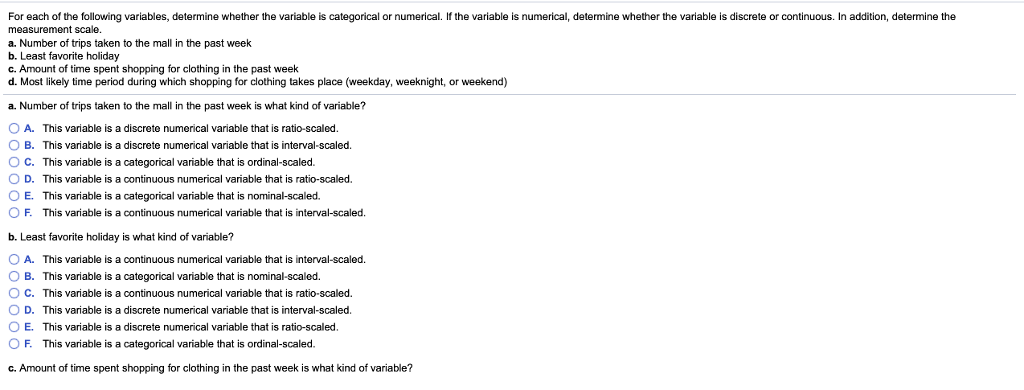For each of the following variables, determine whether the variable is categorical or numerical. If the variable is numerical, determine whether the variable is discrete or continuous. In addition, determine the measurement scale. a. Number of trips taken to the mall in the past week b. Least favorite holiday c. Amount of time spent shopping for clothing in the past week d. Most likely time period during which shopping for clothing takes place (weekday, weeknight, or weekend) a. Number of...

• ### Classify the variables A to F as qualitative or quantitative. For quantitative data, please state whether...

Classify the variables A to F as qualitative or quantitative. For quantitative data, please state whether the variable is discrete or continuous. Then, for each one, distinguish between the four (4) levels of measurement (nominal, ordinal, interval and ratio). What is your Year of Graduation (YOG)? How many courses are you enrolled in? What method of payment do you use to pay for textbooks? Cash Credit Card Debit Card

• ### Classify the variables A to F as qualitative or quantitative. For quantitative data, please state whether...

Classify the variables A to F as qualitative or quantitative. For quantitative data, please state whether the variable is discrete or continuous. Then, for each one, distinguish between the four (4) levels of measurement (nominal, ordinal, interval and ratio). What is the total cost of textbooks for your courses? How many times do you see your Academic Advisor during the semester? How do you rate the Add/Drop period? Very good/ Good/ Bad